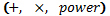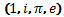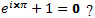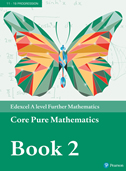Translate

An award winning sixth-form college in the heart of Central London offering a wide range of GCSE and A-Level courses

The quality of the curriculum, teaching and learners' achievements is excellent
ISI INSPECTION REPORT - APRIL 2023

T (+44) 20 7409 7273
Albemarle Independent College, 18 Dunraven Street, Mayfair, London W1K 7FE# FURTHER MATHEMATICS (PEARSON)

A Level Course Outline

What is Further Mathematics?Further Mathematics is a natural extension to Mathematics A Level, taking you twice as far and as deep. The skill set developed in this course prepares you for the material and rigour of university level study in Mathematics and Mathematical Sciences like Physics, Engineering, Economics and Chemistry. In some top universities Further Mathematics is a strong preference for application to mathematical subjects and additional lectures are often necessary for those who have not taken it.

Further Mathematics is accessible to those who excel in their Mathematics A Level, typically but not exclusively those on course for an A grade in it. The units studied are incrementally more challenging both in terms of the material and problem-solving skills previously developed.

What Topics will I study?

In Further Pure Mathematics modules you will:

• Discover what happens when we imagine the square root of a negative number, an idea which has shed light in, for example, the world of Quantum Physics.
• Find out what a matrix is, learn to manipulate and analyse matrices which transform data and space as used in Economics, Sciences and Computing.
• Solve equations that involve differentials used in Maths A Level. These allow you to predict the behaviour of systems in, for example, Biology and Economics.
• Learn how to express functions and solutions as a series of increasing x powers; a powerful approximating tool which aided the idea of electron orbits in chemistry.

To underpin this you will need a strong understanding of the abstract reasoning which has led to these advances, why do the three fundamental mathematical operationslink the four most fundamental constants; Why doesYou have a degree of choice in which applied modules are taken; so if you have a preference for one area you can study it in more depth. In Statistics you learn how to test ideas and claims using the scientific method, and find out how confident you can be in your answers. Mechanics provides you with skills to model motion, forces and stability. Decision Mathematics introduces techniques to plan for optimal efficiency, providing management and leadership skills as well as the logical foundations of computer programming. You may study an additional Further Pure Mathematics module which introduces a larger geometrical toolkit and a variety of integration methods.

 Further Mathematics (EDX) A level SpecificationEach unit has a 1½ hour exam with 75 marks and is equally weighted toward the A Level. Paper 1 and 2 Each 25% Core Mathematics 1: Series, complex numbers, numerical solutions of equations, co-ordinate systems, matrix algebra, proof. Core Mathematics 2: Inequalities, series, first & second order differential equations, further complex numbers, Maclaurin and Taylor Series. Paper 3 and 4 Each 25% (Choose two options) Further Mechanics 1 & 2 The study of forces and motion, building up from Newton's laws in one dimension with a constant force to more dimensions, variable forces and composite bodies. Further Statistics 1 & 2 Starting from the analysis and representation of data, statistics moves towards testing the truth of claims and quantitatively considering bias and the efficiency of methods. Decision Mathematics 1 & 2 Looks to optimise systems, finding the most efficient and suitable method for completing a variety of tasks. Further Pure Mathematics 1 & 2 Further matrix algebra, vectors, hyperbolic functions, differentiation, integration, further co-ordinate systems.

Structure of the Course
This course will be taught for 6x 1hour lessons per week. Homework is set at the end of each session and deadlines for submissions must be met. A full set of notes and examination questions is provided. The UK Senior Mathematics Challenge is offered as an enrichment activity.

 Core Textbooks Edexcel A level Further Mathematics Core Pure Mathematics Book 2 Textbook Recommended Reading Euler: The Master of Us All - William Dunham ISBN-13: 978-0883853283 Letters to a Young Mathematician - Ian Stewart ISBN-13: 978-0465082322